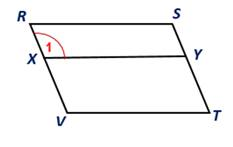Chapter 4.1, Problem 29E### Elementary Geometry for College St...

6th Edition
Daniel C. Alexander + 1 other
ISBN: 9781285195698

#### Solutions

Chapter
Section### Elementary Geometry for College St...

6th Edition
Daniel C. Alexander + 1 other
ISBN: 9781285195698
Textbook Problem
59 views

# In Exercises 27 to 30, use the definition of a parallelogram to complete each proof.Given: Parallelogram RSTV; also X Y - ∥ V T - Prove: ∠ 1 ≅   ∠ S Plan: First show that RSYX is a parallelogram.To determine

To prove:

1 S in the given figure.

Explanation

Given:

Parallelogram RSTV; also XY-VT-.

Corollary:

1. A parallelogram is a quadrilateral in which both pairs of opposite sides are parallel.

2. Opposite angles of a parallelogram are congruent.

Approach:

From the given parallelogram RSTV, and XY-VT-.

Here RS-VT- and XY-VT

### Still sussing out bartleby?

Check out a sample textbook solution.

See a sample solution

#### The Solution to Your Study Problems

Bartleby provides explanations to thousands of textbook problems written by our experts, many with advanced degrees!

Get Started

#### Solve the equations in Exercises 126. 2x3x2x3x+1=0

Finite Mathematics and Applied Calculus (MindTap Course List)

#### 29. Find what value of c is the function a probability density function.

Mathematical Applications for the Management, Life, and Social Sciences

#### Explain why partial counterbalancing is sometimes necessary.

Research Methods for the Behavioral Sciences (MindTap Course List)